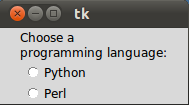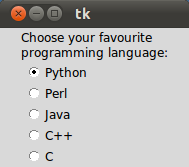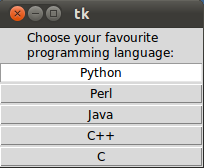A-A+

# [Tkinter Gui 教程05] Radiobutton 控件

2019年12月20日 11:14 阅读 213 views 次

## 简单例子

``````from Tkinter import *

root = Tk()

v = IntVar()

Label(root,
text="""Choose a
programming language:""",
justify = LEFT,
text="Python",
variable=v,
value=1).pack(anchor=W)
text="Perl",
variable=v,
value=2).pack(anchor=W)

mainloop()``````## 改进例子

``````from Tkinter import *

root = Tk()

v = IntVar()
v.set(1)  # initializing the choice, i.e. Python

languages = [
("Python",1),
("Perl",2),
("Java",3),
("C++",4),
("C",5)
]

def ShowChoice():
print v.get()

Label(root,
programming language:""",
justify = LEFT,

for txt, val in languages:
text=txt,
variable=v,
command=ShowChoice,
value=val).pack(anchor=W)

mainloop()``````## 指示器

``````    Radiobutton(root,
text=txt,
indicatoron = 0,
width = 20,
variable=v,
command=ShowChoice,
value=val).pack(anchor=W)``````## 相关tkinter 教程系列

### 布施恩德可便相知重

#### 微信扫一扫打赏#### 支付宝扫一扫打赏×